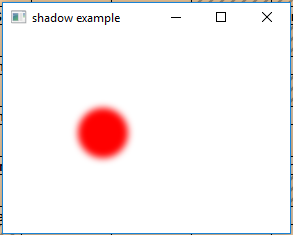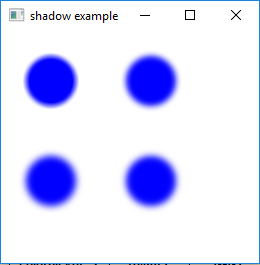Shadow class is a part of JavaFX. Shadow class creates a monochromatic shadow with blurry edges. The Shadow is of black Color (by default) and can be combined with the original to create a shadow. The Shadow of different color can be added with original to create a Glow effect. Shadow class inherits Effect class.

Constructors of the class:

2. Shadow(BlurType t, Color c, double r): Creates a new Shadow Object with specified blur type, color, and radius.

Commonly Used Methods:

Method Explanation
getBlurType() Returns the blur type of the effect.
getColor() Returns the color of the effect.
getInput() Returns the value of property input.
setBlurType(BlurType v) Sets the blur type of the Shadow effect.
setColor(Color v) Sets the Color of the Shadow effect.
setInput(Effect v) Sets the value of the property input.

Below programs illustrate the use of Shadow class:

1. Java program to create a Circle and add Shadow effect to it: In this program we will create a Circle named circle and create a Shadow effect shadow with specified radius and color. The shadow effect will be added to the circle using the setEffect() function and the circle will be added to the group. The circles will be translated to specific position in the stage using setTranslateX() and setTranslateY() function. The group will be added to the scene and the scene will be added to the stage.

 `// Java program to create a Circle  ` `// and add Shadow effect to it ` `import` `javafx.application.Application; ` `import` `javafx.scene.Scene; ` `import` `javafx.scene.control.*; ` `import` `javafx.scene.layout.*; ` `import` `javafx.stage.Stage; ` `import` `javafx.scene.image.*; ` `import` `javafx.scene.effect.*; ` `import` `java.io.*; ` `import` `javafx.scene.shape.Circle; ` `import` `javafx.scene.paint.Color; ` `import` `javafx.scene.Group; ` ` `  `public` `class` `shadow_1 ``extends` `Application { ` ` `  `    ``// launch the application ` `    ``public` `void` `start(Stage stage) ``throws` `Exception ` `    ``{ ` ` `  `        ``// set title for the stage ` `        ``stage.setTitle(``"shadow example"``); ` ` `  `        ``// create a circle ` `        ``Circle circle = ``new` `Circle(``50``.0f, ``50``.0f, ``25``.0f); ` ` `  `        ``// translate to a position ` `        ``circle.setTranslateX(``50``.0f); ` `        ``circle.setTranslateY(``50``.0f); ` ` `  `        ``// create a shadow effect ` `        ``Shadow shadow = ``new` `Shadow(``10``, Color.RED); ` ` `  `        ``// set effect ` `        ``circle.setEffect(shadow); ` ` `  `        ``// create a Group ` `        ``Group group = ``new` `Group(circle); ` ` `  `        ``// create a scene ` `        ``Scene scene = ``new` `Scene(group, ``200``, ``200``); ` ` `  `        ``// set the scene ` `        ``stage.setScene(scene); ` ` `  `        ``stage.show(); ` `    ``} ` ` `  `    ``// Main Method ` `    ``public` `static` `void` `main(String args[]) ` `    ``{ ` ` `  `        ``// launch the application ` `        ``launch(args); ` `    ``} ` `} `

Output:`// Java program to create four Circles and add Shadow ` `// effect to it which are of different BlurType ` `import` `javafx.application.Application; ` `import` `javafx.scene.Scene; ` `import` `javafx.scene.control.*; ` `import` `javafx.scene.layout.*; ` `import` `javafx.stage.Stage; ` `import` `javafx.scene.image.*; ` `import` `javafx.scene.effect.*; ` `import` `java.io.*; ` `import` `javafx.scene.shape.Circle; ` `import` `javafx.scene.paint.Color; ` `import` `javafx.scene.Group; ` ` `  `public` `class` `shadow_2 ``extends` `Application { ` ` `  `    ``// launch the application ` `    ``public` `void` `start(Stage stage) ``throws` `Exception ` `    ``{ ` ` `  `        ``// set title for the stage ` `        ``stage.setTitle(``"shadow example"``); ` ` `  `        ``// create a circle ` `        ``Circle circle = ``new` `Circle(``0``.0f, ``0``.0f, ``25``.0f); ` `        ``Circle circle1 = ``new` `Circle(``0``.0f, ``0``.0f, ``25``.0f); ` `        ``Circle circle2 = ``new` `Circle(``0``.0f, ``0``.0f, ``25``.0f); ` `        ``Circle circle3 = ``new` `Circle(``0``.0f, ``0``.0f, ``25``.0f); ` ` `  `        ``// translate to a position ` `        ``circle.setTranslateX(``50``.0f); ` `        ``circle.setTranslateY(``50``.0f); ` ` `  `        ``// translate to a position ` `        ``circle1.setTranslateX(``150``.0f); ` `        ``circle1.setTranslateY(``50``.0f); ` ` `  `        ``// translate to a position ` `        ``circle2.setTranslateX(``50``.0f); ` `        ``circle2.setTranslateY(``150``.0f); ` ` `  `        ``// translate to a position ` `        ``circle3.setTranslateX(``150``.0f); ` `        ``circle3.setTranslateY(``150``.0f); ` ` `  `        ``// create shadow effect ` `        ``Shadow shadow1 = ``new` `Shadow(BlurType.values()[``0``], Color.BLUE, ``10``); ` `        ``Shadow shadow2 = ``new` `Shadow(BlurType.values()[``1``], Color.BLUE, ``10``); ` `        ``Shadow shadow3 = ``new` `Shadow(BlurType.values()[``2``], Color.BLUE, ``10``); ` `        ``Shadow shadow4 = ``new` `Shadow(BlurType.values()[``3``], Color.BLUE, ``10``); ` ` `  `        ``// set effect ` `        ``circle.setEffect(shadow1); ` `        ``circle1.setEffect(shadow2); ` `        ``circle2.setEffect(shadow3); ` `        ``circle3.setEffect(shadow4); ` ` `  `        ``// create a Group ` `        ``Group group = ``new` `Group(circle, circle1, circle2, circle3); ` ` `  `        ``// create a scene ` `        ``Scene scene = ``new` `Scene(group, ``200``, ``200``); ` ` `  `        ``// set the scene ` `        ``stage.setScene(scene); ` ` `  `        ``stage.show(); ` `    ``} ` ` `  `    ``// Main Method ` `    ``public` `static` `void` `main(String args[]) ` `    ``{ ` ` `  `        ``// launch the application ` `        ``launch(args); ` `    ``} ` `} `

Output:Note: The above programs might not run in an online IDE. Please use an offline compiler.

Don’t stop now and take your learning to the next level. Learn all the important concepts of Data Structures and Algorithms with the help of the most trusted course: DSA Self Paced. Become industry ready at a student-friendly price.

My Personal Notes arrow_drop_upCheck out this Author's contributed articles.

If you like GeeksforGeeks and would like to contribute, you can also write an article using contribute.geeksforgeeks.org or mail your article to contribute@geeksforgeeks.org. See your article appearing on the GeeksforGeeks main page and help other Geeks.

Please Improve this article if you find anything incorrect by clicking on the "Improve Article" button below.

Article Tags :
Practice Tags :

1

Please write to us at contribute@geeksforgeeks.org to report any issue with the above content.